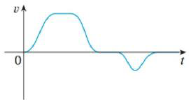Chapter 4.9, Problem 54E### Single Variable Calculus: Early Tr...

8th Edition
James Stewart
ISBN: 9781305270343

#### Solutions

Chapter
Section### Single Variable Calculus: Early Tr...

8th Edition
James Stewart
ISBN: 9781305270343
Textbook Problem

# The graph of the velocity function of a particle is shown in the figure. Sketch the graph of a position function.To determine

To sketch: The graph of a position function for the given velocity function.

Explanation

Given Data:

Refer to the Figure in the textbook for the given velocity function v(t) .

Write the expression for velocity function v(t) in terms of position function r(t) as follows.

v(t)=ddt[r(t)]

Integrate on both sides of the expression as follows.

v(t)dt={ddt[r(t)]}dt=r(t)

Rewrite the expression as follows

### Still sussing out bartleby?

Check out a sample textbook solution.

See a sample solution

#### The Solution to Your Study Problems

Bartleby provides explanations to thousands of textbook problems written by our experts, many with advanced degrees!

Get Started

#### Expand each expression in Exercises 122. (4y2)y

Finite Mathematics and Applied Calculus (MindTap Course List)

#### solve the equation by using the quadratic formula. 137. 8x2 8x 3 = 0

Applied Calculus for the Managerial, Life, and Social Sciences: A Brief Approach

#### In Problems 19-44, factor completely. 32.

Mathematical Applications for the Management, Life, and Social Sciences

#### The graph at the right has equation:

Study Guide for Stewart's Multivariable Calculus, 8th

#### If , then h′(x) = 8t3 + 2t 24x2 + 2 8x3 + 2x – 3 8x3 + 2x

Study Guide for Stewart's Single Variable Calculus: Early Transcendentals, 8th